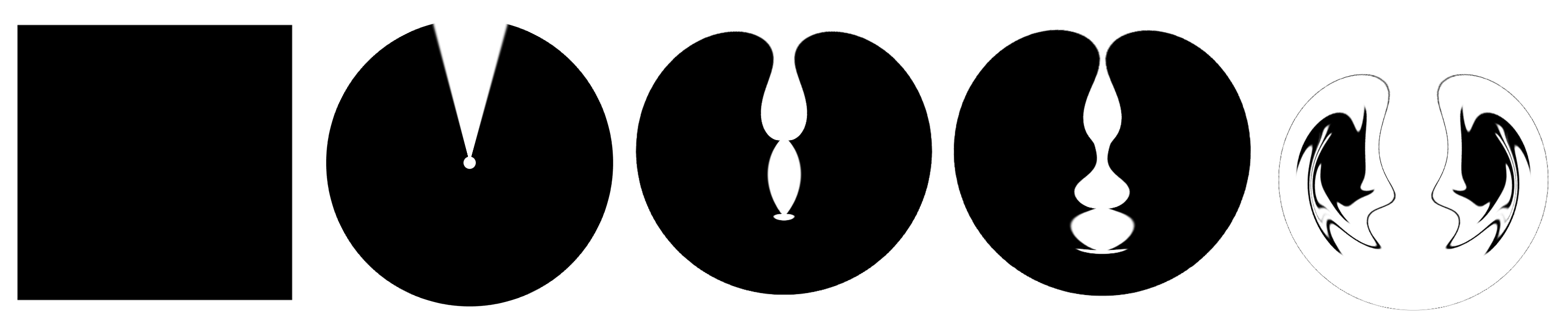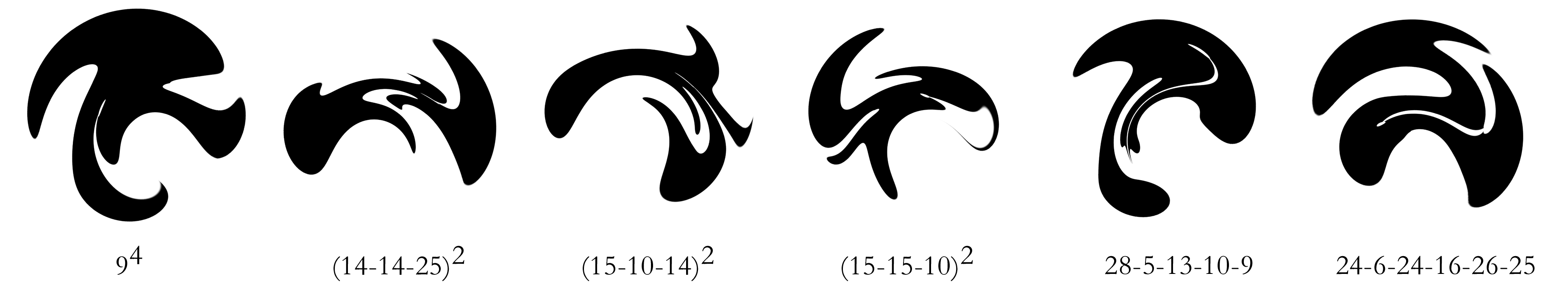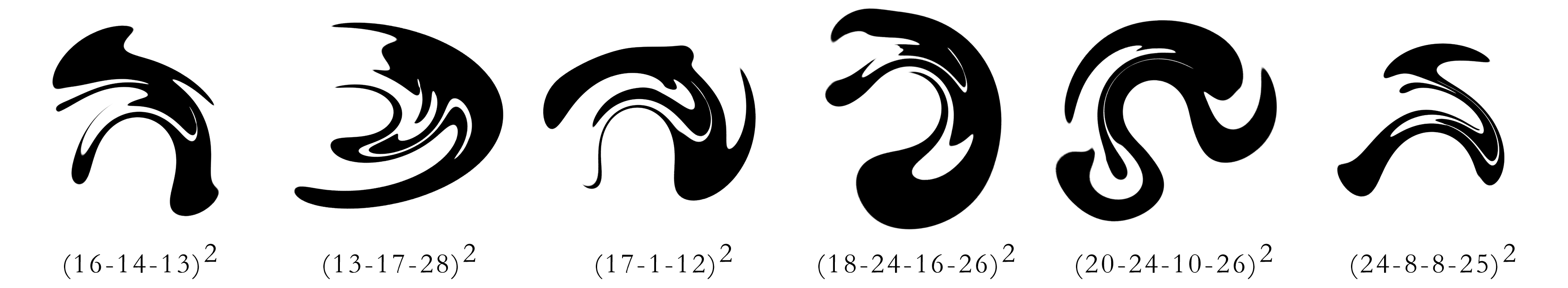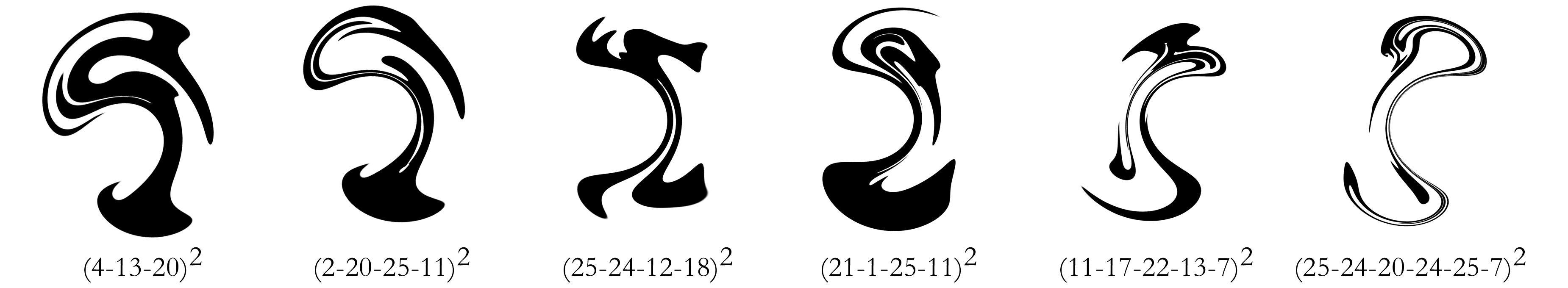Serial Polar Transformations Of Simple Geometries

Leo S. Bleicher

San Diego, CA 92037

Porterbleicher.g2gm.net/computed-paintings

Abstract

Complex forms can be generated by application of geometric transformations to elementary shapes and tessellations. The polar coordinate conversion used in conjunction with standard symmetry operations creates unusual results. The appearance of these forms is dependent on the starting shape, the operations performed, and the order of those operations, giving rise to a wide variety of images. In this paper serial application of geometric transformation operations to a square is explored.

Introduction

Geometric transformation of shapes to obtain visually interesting or mathematically useful results is a common process. Use of the standard symmetry operations of rotation, translation and reflection in the creation of patterns and tilings is one of the most ancient decorative methods. While an infinite variety of beautiful and useful patterns can be generated using these techniques, there are many possibilities not accessible to this method. Tilings of greater complexity have been created, notably in the work of M. C. Escher and V. Vasserelli, in which the shape and size of the design elements are modified based on their location in the design. Computationally, these types of shapes and tilings can be generated by geometric transformations of simpler tessellations. In this paper complex forms will be constructed through serial application of geometric transformations to elementary shapes. Central to this process will be the use of the polar coordinate conversion.

The Polar Transformation

The polar transformation is a well known and widely used mathematical operation. The standard implementation of this transformation converts Cartesian coordinates using horizontal and vertical axes to polar coordinates using radius and angle. The effect of this operation is to convert horizontal lines to circles and vertical lines to rays radiating from the origin. The polar operation can be expressed mathematically as [x, y ®[xsin y, xcos y]. While rotation, reflection and translation have the property of preserving distance relationships between any two points in the starting object, the polar transformation does not preserve these topological relationships. However, neighboring points remain neighbors after transformation and the genus of the shape remains unchanged.

Transformed Tilings

Execution of a geometric transformation on a simple tessellation of the plane gives a visual sense of the effect of the operation. Application of some well known coordinate transformations to a plane tessellated by squares leads to tessellations difficult to obtain in other ways, as shown in Figure 1. Example 1b shows the polar transformation, 1c shows the inverse polar transformation where [x, y ® [Ö(x2+y2), atan(y/x)]. The tiling in Figure 1d has been displaced by a sine function: [x, y] ® [x+asin(x/p), y+asin(y/p)] where p is the period of the wave and a the amplitude . Figure 1e shows the application of circle inversion: [x, y] ®[(xr2)/(x2 +y2), (yr2)/(x2+y2)) where r is the radius of the inverting circle . The final image in the figure, 1f, shows the results of two applications of the polar transformation. Formally, this transformation can be written as [x, y] ® [sin(xcos y)(xsin y), cos(xcos y)(xsin y)], however, it is simpler to treat this operation as serial steps if one is to carry on with further transformation operations.Figure 1a-f: Transformed tilings.

As the transformation sequences become more complex than the double polar transformation shown in Figure 1f, visualization of transformed tessellations becomes less informative and more taxing. A general impression of the effect of the transformation can be obtained by operation on a simple shape such as a square. In this representation two applications of the polar transformation generates 2c. The balance of Figure 2 shows (2a) the starting point for these geometrically transformed images; 2b, the result of a single polar transformation; 2d, three polar transformations applied; and 2e, shows an approximation of the limit case: ten polar transformation operations applied serially.Figure 2a-e: Serial polar coordinate transformations of a square.

Serial Geometric Transformations of Squares

Addition of the standard symmetry operations to these more complex geometric transformation operations yields a palate of processes which can be combined to create interesting and unusual shapes. In particular, the combination of the polar transformation with rotations rapidly yields images like those shown in Figure 3. Each image in Figure 3 is the product of a series of seven transformations of a square. These transformation series each begin with a polar transformation to give 2b, this is followed by three operations chosen from Table 1 interleaved with polar transformations and completed with a final polar transformation. The captions on the Figures indicate which operations from Table 1 were used to create each image.

 Operation # Operation description 1-11 Rotate (n · 30°) 12 Mirror Horizontal

The images in Figure 3 were selected from the 1728 possible combinations of three operations from Table 1. Fewer than 1000 of these are unique under the standard symmetry operations of rotation and reflection. However, the order of operations is critical, as exemplified by the sequences 3-10-8 and 8-3-10 (see rows 3 & 4 of Figure 3). Remarkably, in as few as four transformations (the polar, rotate sequence can be easily recast as a single transformation) a geometrically simple object such as a square can be transformed into a complex shape evocative of natural forms.Figure 3

There are four approaches to expanding the range of possible shapes that can be generated using this method of serial geometric transformations. Use of starting shapes other than the square, or partitioning the square into tiles as in Figure 1, can radically change the appearance of the final image generated. Addition of novel transformation types to the collection presented in Table 1 allows exploration of different topological spaces. Use of smaller rotation increments allows interpolation between the images generated from this process. Fine grained interpolation of the angles of rotation creates movies illustrating the gradual progression of the image generated as the angle of rotation is incremented. Finally, allowing more steps in the sequence of geometric transformations allows greater complexity in the results. Of course, exhaustive enumeration and review of each image rapidly becomes impractical.

 Operation # Operation Description 1-23 Rotate (n · 15°) 24 Horizontal Reflection 25 Vertical Reflection 26 Circle Inversion 27 Translate Left 28 Translate Right

A program to generate images based on an expanded selection of operations (Table 2) was written to explore the possibilities of serial geometric transformations. A variety of methods were used to generate transformation sequences including random, weighted and genetic algorithms. The transformation sequences generated ranged from 5 to 13 operations, interleaving the polar transform after each selection from Table 2, with optional repetition of the entire sequence. Again, a square was the starting point for each image. This expansion of the number and types of operations leads to an explosion in the number of possible images. Using 6 transformations chosen from 28 types yields one billion possible images. The images presented in Figure 4 were selected from many thousands generated and reviewed. The captions on the figures detail which operations from Table 2 were used to create each image, with superscripts indicating repetition.

Observations

The creation of images resulting from serial polar and geometric transformations would be nearly impossible without powerful computational devices. Formulation of transformations which would be hideously complex if done in one step, as sequences of relatively simple operations allows these images to be conceived. Though the image resulting from a sequence of transformations is completely determined by that sequence, the esthetic impact of that image can not be predicted.

The images in Figure 4 highlight the richness of forms accessible through the use of serial polar and geometric transformations. The polar transforms distortion of distance relationships and relative scales within the transformed object gives rise to the distinctive appearance of these images. The balance and grace of these constructions belies their origin as squares transformed by simple formulas. Severely stretched filaments extruded from the edges of the original square recall the dynamic quality of quick brush strokes. Interestingly, many of the most attractive images are the product of repeated sequences of operations. Serial geometric transformations have possibilities far beyond the scope of this work and, even with the methods outlined here, many more interesting forms await discovery.Figure 4: Transformations of a square using sequences of 9 to 25 operations.

References

 Paintings using this type of coordinate transformation see: Peden, D. D. 2000. "Gridfield form and patterning". Second Annual Conference of the International Society of the Arts, Mathematics, and Architecture. Albany, N.Y. June. Reviewed in http://www.maa.org/mathland/mathtrek_8_14_00.html

 Krawczyk, R. J. Curving Spirolaterals, Mathematics & Design, 2001